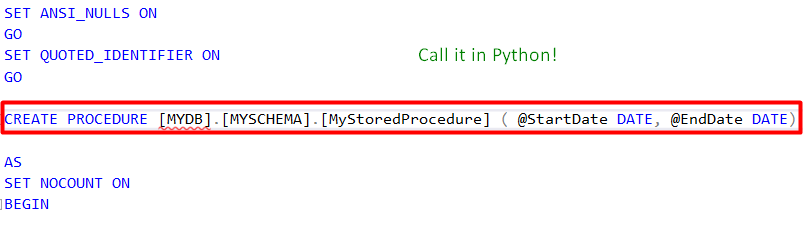# Pandas Read_Sql With Variable Parameters — Alternative Way

## Call MS SQL Stored Procedure with Parameters in PythonStored Procedure Creation by Ayşe Kübra Kuyucu

This is something we need so many times. You have your Stored Procedure (SP) and, want to call it in Python.

And, generally, an SP has its parameters and these parameters need to be variables most of the time.

Indeed, this issue is discussed and solved in Stack overflow. You can check it here. However, I think there is a better way doing this.

First, we need our SP. In this example, name of my SQL — related things are below.

Database: MYDB

Schema: MYSCHEMA

Stored Procedure: MyStoredProcedure

In this example, our SP is taking 2 date parameters: StartDate and EndDate.

Here is how I created my SP in MS SQL:

``````CREATE PROCEDURE [MYDB].[MYSCHEMA].[MyStoredProcedure] ( @StartDate DATE, @EndDate DATE)
``````

Here is how I call that SP in MS SQL:

``````EXECUTE [MYDB].[MYSCHEMA].[MyStoredProcedure] @StartDate='20221001', @EndDate='20221101'
``````

Now, we are ready to write our query in Python.

We will use an awesome library: Pandas. So, import it.

``````import pandas as pd
``````

Then, we need to define our date variables:

``````start_date = '20221001'
end_date = '20221101'
``````

They are in string format and, exactly same as we write them in MS SQL.

Now we can write our query:

``````query = 'EXECUTE [MYDB].[MYSCHEMA].[MyStoredProcedure] @StartDate=?, @EndDate=?'
``````

For the parameters part, all you need is to put ‘=?’ at the and of parameter names as a difference from SQL native code.

My SP is selecting some columns so, I want to put the output into a DataFrame.

Call SP in Python:

``````df = pd.read_sql(query, engine, params=(start_date, end_date))
``````

You can do the same thing with another function of Pandas: `read_sql_query`

``````df = pd.read_sql_query(query, engine, params=(start_date, end_date))
``````

So, we created an SP with date parameters, called it in MS SQL and Python. (For other variable types, you can do the same thing.)

Hope you enjoyed and benefited from it.